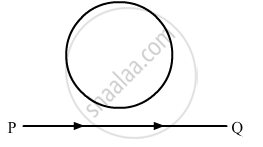# Depict the Direction of the Current Induced in the Loop When the Current in the Wire Pq is Constantly Increasing. - Physics

A conducting loop is held above a current carrying wire PQ as shown in the figure. Depict the direction of the current induced in the loop when the current in the wire PQ is constantly increasing.#### Solution

The increasing magnetic field in the loop due to wire PQ is out of the plane of the paper (perpendicular to the plane). So, the direction of the induced current in the loop will be such that it produces an inward magnetic field (perpendicular to the plane). Thus, the current induced in the loop is in clockwise direction (using the right-hand thumb rule).

Concept: Magnetic Force
Is there an error in this question or solution?